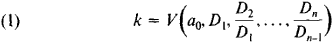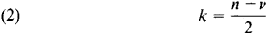# Routh-Hurwitz Problem

The following article is from The Great Soviet Encyclopedia (1979). It might be outdated or ideologically biased.

## Routh-Hurwitz Problem

the problem of determining the number k of roots of an algebraic equation

a0Zn + a1Zn-1 + … + an-1z + an = 0

with positive real parts. If the coefficients a0, a1,..... an are real, thenwhere V is the number of changes of sign in the series of numbers a0, D1, D2/D1,…. and the Dl (l = 1, 2, …, n) are the Hurwitz determinants (seeHURWITZ CRITERION). The special cases where some of the Dl vanish must be treated separately. If l = 1, the Hurwitz criterion follows from equation (1).

Equation (1) was established by the German mathematician A. Hurwitz in 1895. Earlier studies of the Routh-Hurwitz problem using other approaches were made by the French mathematician C. Hermite in 1856 and the British mathematician E. Routh in 1877. Routh found a special algorithm for the computation of k.

Equation (1) can be replaced by a geometric rule. Thus, consider the complex quantity

a0(iω)n + a1 (iω)n-1 + … + an

As ω varies from 0 to + ∞, the point representing this quantity describes a curve. If in the process the argument θ of the point increases by Δθ = vπ/2, thenThe special case where the curve passes through the origin must be treated separately. If k= 0, it follows from equation (2) that v = n, which yields A. Mikhailov’s stability criterion. The Mikhailov criterion was established in 1939 and is widely used in engineering literature.

Generalizations of the Routh-Hurwitz problem to the case of complex coefficients a0, a1,…, an and to the case of transcendental equations are encountered in applications.